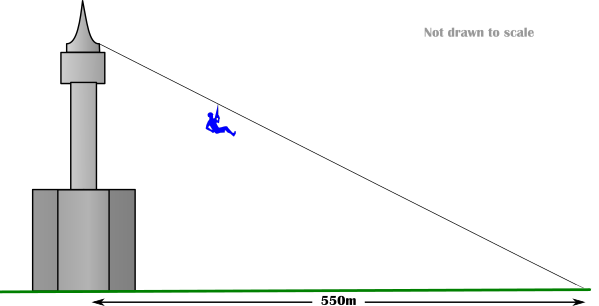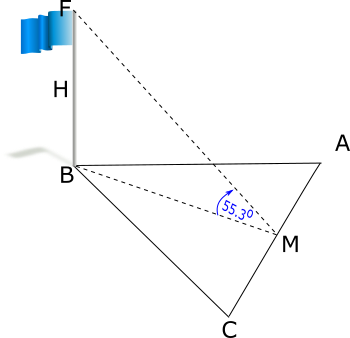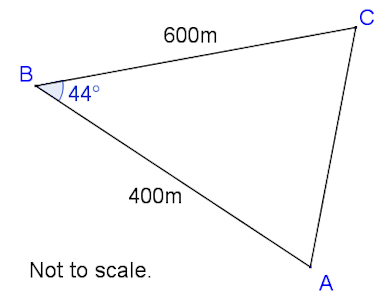# Angles of Elevation and Depression

## Problems adapted from questions set for previous Mathematics exams.

### 1.

GCSE Higher

An arborist sights the top of a tree using a clinometer and reads the angle of elevation to be 29o. Her clinometer is 28 metres from the base of the tree and is on a tripod making it 1.5 metres above ground level.

This diagram is not drawn to scale.Calculate the full height of the tree.

### 2.

GCSE Higher

Taptaya Park Tower is over two hundred metres tall. There is a zip wire from the observation deck to a point on the ground 550 metres away in a northerly direction.(a) If the beginning of the zip wire is 210 metres above the ground find the angle of depression of the end point of the zip wire as seen from the beginning.

It is decided that the zip wire is slightly too steep so the end point is moved so that it is now further away.

(b) Find the horizontal distance if the angle of elevation of the beginning of the zip wire as seen from the end is now 18o.

### 3.

IB Studies

Neung is at Bangkok's Suvarnabhumi Airport watching the planes take off. He observes a plane that is at an angle of elevation of 25o from where he is standing at point N. The plane is at a height of 390 metres as can be seen in the following diagram (not to scale).(a) Calculate the horizontal distance, NH, of the plane from Neung. Give your answer to the nearest metre.

The plane took off from a point T, which is 230 metres from where Neung is standing, as shown in the following diagram (not to scale).(b) Calculate the angle ATH, the take-off angle of the plane to the nearest degree.

### 4.

IB Studies

ABC is a triangular car park on horizontal ground. The length of AB is 90m and the length of AC is 65m. The size of angle BCA is 68o.

(a) Find the size of angle ABC.

(b) Find the area of the triangular car park.

M is the midpoint of AC.

(c) Find the length of BM.

A vertical flag pole, FB, is erected in one corner of the car park with its base at B.The angle of elevation of F from M is 55.3o. H is the midpoint of the flag pole.

(d) Calculate the angle of elevation of H from M.

### 5.

IB Studies

A drone flying test course is in the shape of a triangle, ABC, with AB = 400m , BC = 600m and angle ABC = 44o. The course starts and finishes at point A.(a) Calculate the total length of the course to the nearest metre.

It is estimated that a drone can travel at an average speed of 4.5ms–1.

(b) Calculate an estimate of the time taken to fly around the course. Give your answer to the nearest minute.

(c) Find the size of angle ACB.

To comply with safety regulations, the area inside the triangular course must be kept clear of people, and the shortest distance from B to AC must be greater than 275 metres.

(d) Calculate the area that must be kept clear of people.

(e) Determine, giving a reason, whether the course complies with the safety regulations.

The course is viewed from a tower which rises vertically from point A. The top of the tower is point T. The angle of elevation of T from B is 8o.

(f) Calculate the vertical height, AT , of the tower.

If you would like space on the right of the question to write out the solution try this Thinning Feature. It will collapse the text into the left half of your screen but large diagrams will remain unchanged.

The exam-style questions appearing on this site are based on those set in previous examinations (or sample assessment papers for future examinations) by the major examination boards. The wording, diagrams and figures used in these questions have been changed from the originals so that students can have fresh, relevant problem solving practice even if they have previously worked through the related exam paper.

The solutions to the questions on this website are only available to those who have a Transum Subscription.

Exam-Style Questions Main Page

Search for exam-style questions containing a particular word or phrase:

To search the entire Transum website use the search box in the grey area below.